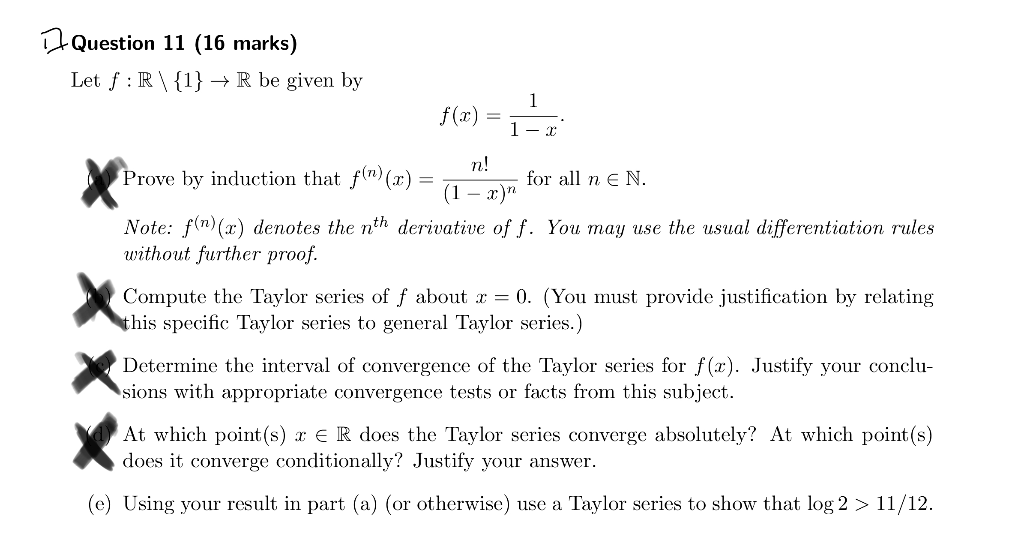# Solved: Question 11 (16 Marks) Let F R\1} -> R Be Given By F(r) = N! Prove By Induction That F(n)(r) E N. For All = (1 - X)" Note: Fn)(x) Denotes The Nth Derivative Of F. You May Use The Usual Differe

By |do e only, in details please.

Question 11 (16 marks) Let f R\1} -> R be given by f(r) = n! Prove by induction that f(n)(r) E N. for all = (1 - x)" Note: fn)(x) denotes the nth derivative of f. You may use the usual differentiation rules without further proof Compute the Taylor series of f about r = 0. (You must provide justification by relating this specific Taylor series to general Taylor series.) Determine the interval of convergence of the Taylor series for f(x). Justify your conclu- sions with appropriate convergence tests or facts from this subject At which point(s) xE R does the Taylor series converge absolutely? At which point(s) does it converge conditionally? Justify your (e) Using your result in part (a) (or otherwise) use a Taylor series to show that log 2 > 11/12